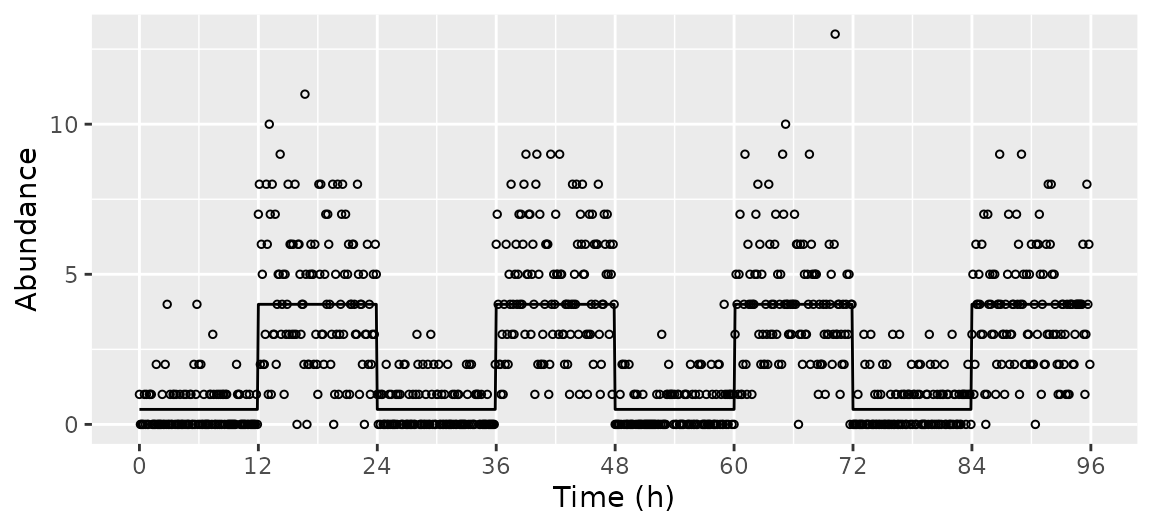library('data.table')
library('ggplot2')
library('simphony')

## Evenly-spaced timepoints

featureGroups = data.table(amp = c(0, 3, 6), phase = c(0, 0, 6),
rhyFunc = c(cos, cos, sin), fracFeatures = c(0.1, 0.8, 0.1))

simData = simphony(featureGroups)

mergedData = mergeSimData(simData)
expectedTrend = getExpectedAbund(simData$featureMetadata, times = seq(0, 48, 0.25)) plotFeatures = c('feature_01', 'feature_05', 'feature_10') ggplot() + facet_wrap(~ feature) + geom_line(data = expectedTrend[feature %in% plotFeatures], aes(x = time, y = mu)) + geom_point(data = mergedData[feature %in% plotFeatures], aes(x = time, y = abund)) + scale_x_continuous(breaks = seq(0, 48, 8)) + labs(x = 'Time (h)', y = 'Abundance')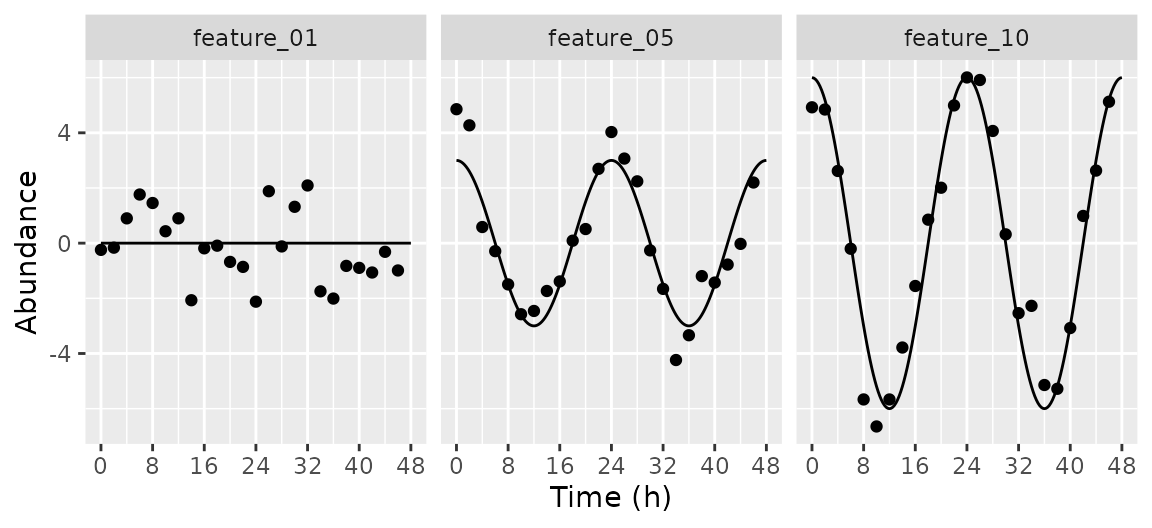ggplot() + geom_point(data = simData$featureMetadata, aes(x = factor(amp0), y = factor(feature))) +
labs(x = 'Amplitude', y = 'Feature')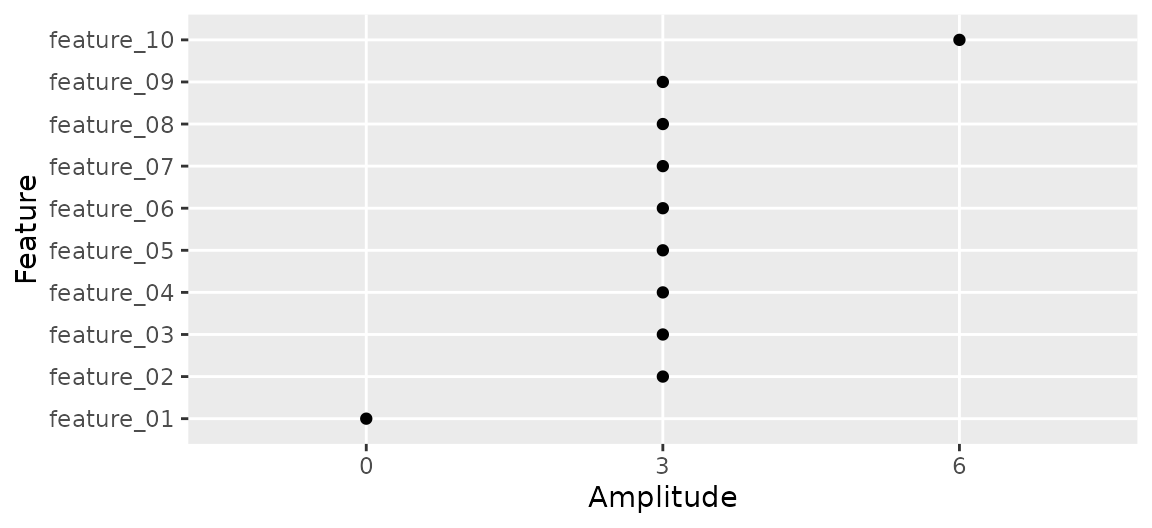## Custom rhythm function

featureGroups = data.table(amp = 6, phase = -3, rhyFunc = function(x) sign(sin(x)))

simData = simphony(featureGroups)

mergedData = mergeSimData(simData)
expectedTrend = getExpectedAbund(simData$featureMetadata, times = seq(0, 48, 0.1)) ggplot() + geom_line(data = expectedTrend[feature %in% plotFeatures], aes(x = time, y = mu)) + geom_point(data = mergedData[feature %in% plotFeatures], aes(x = time, y = abund)) + scale_x_continuous(breaks = seq(0, 48, 8)) + labs(x = 'Time (h)', y = 'Abundance')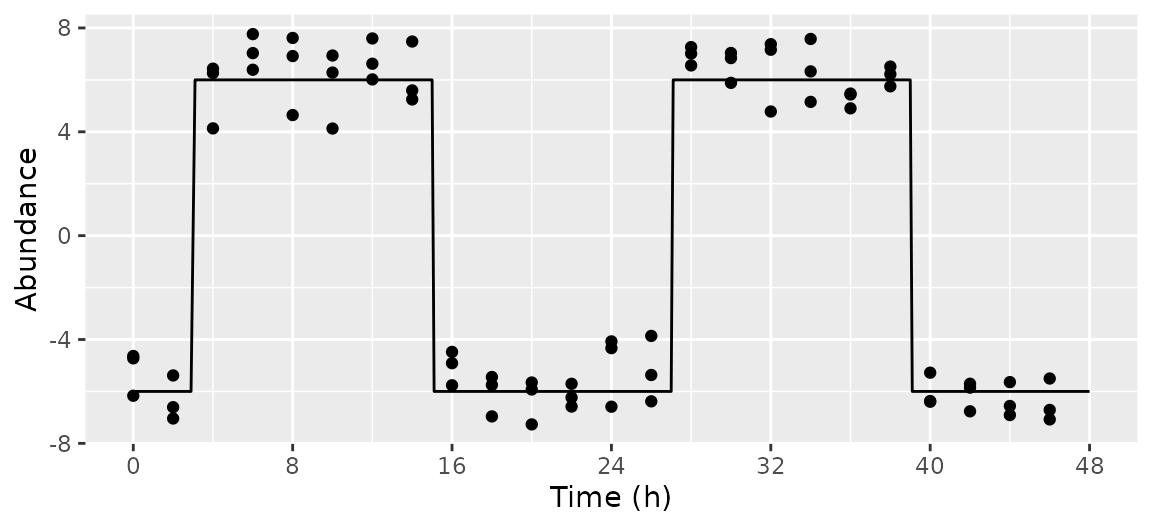## Specified timepoints featureGroups = data.table(amp = c(0, 3)) simData = simphony(featureGroups, timepointsType = 'specified', nFeatures = nrow(featureGroups), timepoints = c(seq(0, 22, 2), seq(24, 42, 6))) mergedData = mergeSimData(simData) expectedTrend = getExpectedAbund(simData$featureMetadata, times = seq(0, 48, 0.25))

ggplot() +
facet_wrap(~ feature) +
geom_line(data = expectedTrend, aes(x = time, y = mu)) +
geom_point(data = mergedData, aes(x = time, y = abund)) +
scale_x_continuous(breaks = seq(0, 48, 8)) +
labs(x = 'Time (h)', y = 'Abundance')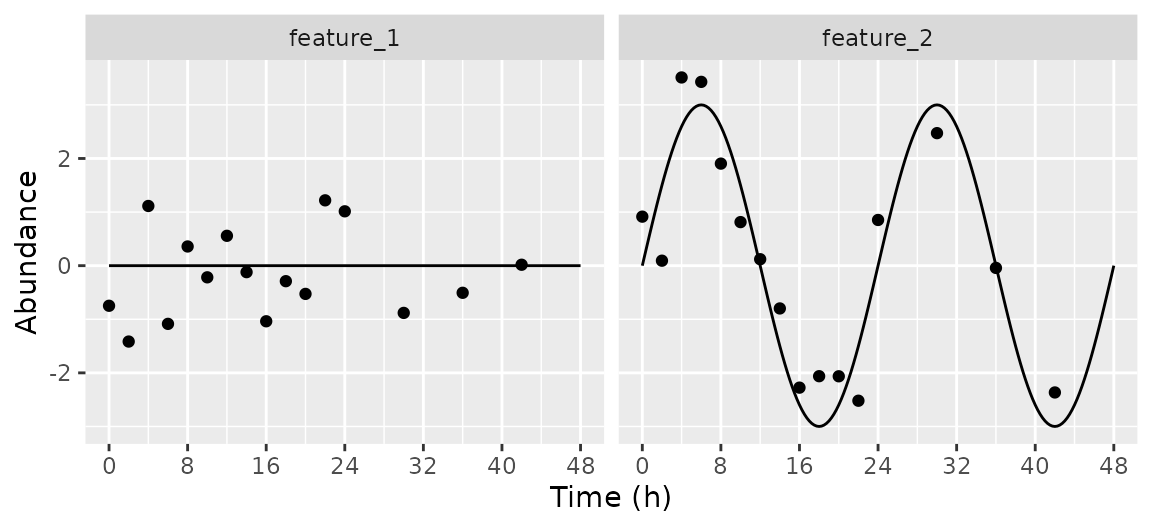## Random timepoints

featureGroups = data.table(amp = c(0, 3))

simData = simphony(featureGroups, timepointsType = 'random', nFeatures = nrow(featureGroups),
timeRange = c(0, 24), nSamplesPerCond = 20)

mergedData = mergeSimData(simData)
expectedTrend = getExpectedAbund(simData$featureMetadata, times = seq(0, 24, 0.25)) ggplot() + facet_wrap(~ feature) + geom_line(data = expectedTrend, aes(x = time, y = mu)) + geom_point(data = mergedData, aes(x = time, y = abund)) + scale_x_continuous(breaks = seq(0, 24, 4)) + labs(x = 'Time (h)', y = 'Abundance')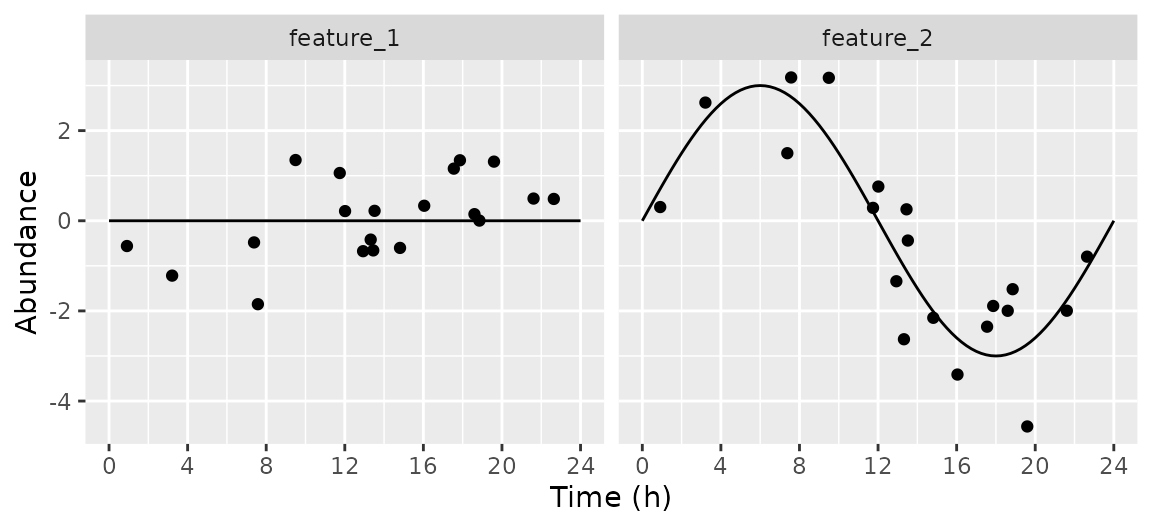## Time-dependent amplitude and base featureGroups = data.table(amp = c(function(tt) 3, function(tt) 3 * 2 ^ (-tt / 24)), base = c(function(tt) tt / 12, function(tt) 0)) simData = simphony(featureGroups, nFeatures = nrow(featureGroups)) mergedData = mergeSimData(simData) expectedTrend = getExpectedAbund(simData$featureMetadata, times = seq(0, 48, 0.25))

ggplot() +
facet_wrap(~ feature) +
geom_line(data = expectedTrend, aes(x = time, y = mu)) +
geom_point(data = mergedData, aes(x = time, y = abund)) +
scale_x_continuous(breaks = seq(0, 48, 8)) +
labs(x = 'Time (h)', y = 'Abundance')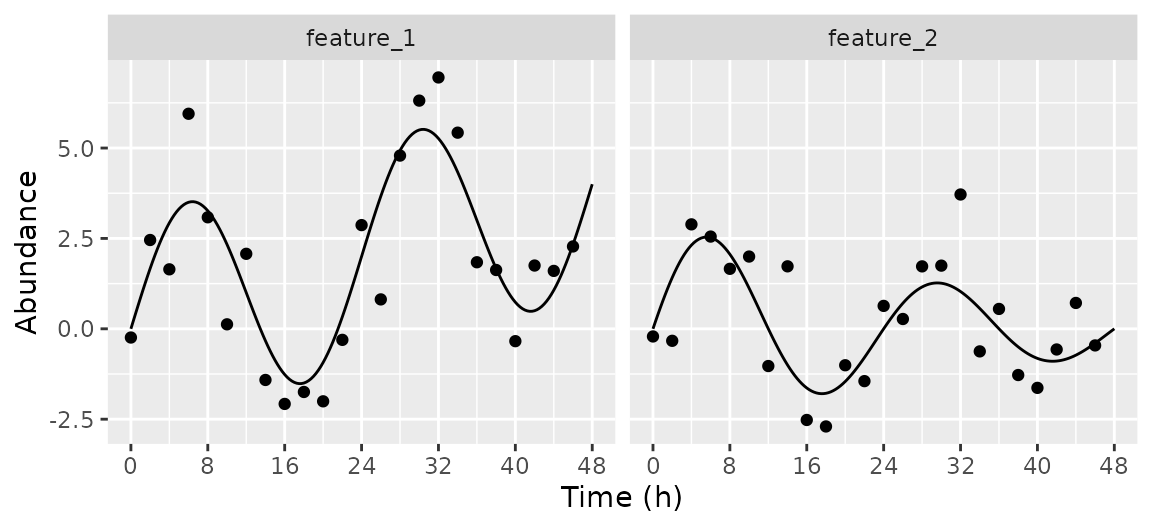## Differential rhythmicity between conditions

featureGroupsList = list(
data.table(amp = c(1, 2, 2), phase = c(0, -3, 0), period = c(24, 24, 22)),
data.table(amp = c(3, 2, 2), phase = c(0,  3, 0), period = c(24, 24, 26)))

simData = simphony(featureGroupsList, nFeatures = nrow(featureGroupsList[]))

mergedData = mergeSimData(simData)
expectedTrend = getExpectedAbund(simData$featureMetadata, times = seq(0, 48, 0.25)) ggplot() + facet_grid(cond ~ feature) + geom_line(data = expectedTrend, aes(x = time, y = mu)) + geom_point(data = mergedData, aes(x = time, y = abund), size = 0.5) + scale_x_continuous(breaks = seq(0, 48, 8)) + labs(x = 'Time (h)', y = 'Abundance')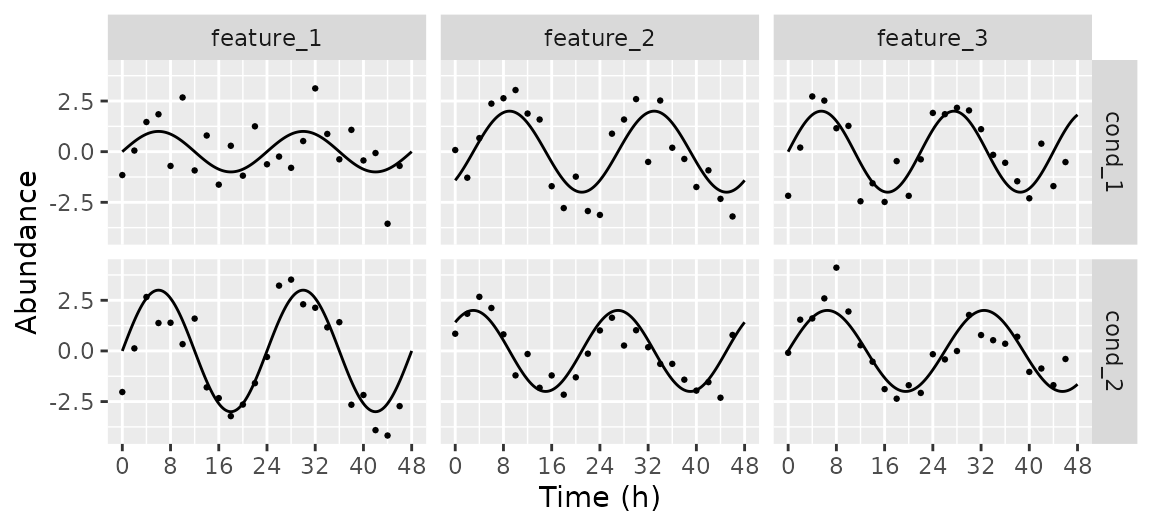## Controlling negative binomial dispersion and base featureGroups = data.table(amp = 3, base = c(4, 6, 8)) dispFunc = function(x) 3 * defaultDispFunc(x) simData = simphony(featureGroups, interval = 4, nFeatures = nrow(featureGroups), family = 'negbinom', dispFunc = dispFunc) mergedData = mergeSimData(simData) expectedTrend = getExpectedAbund(simData$featureMetadata, times = seq(0, 48, 0.25))

ggplot(expectedTrend) +
facet_wrap(. ~ factor(base0)) +
geom_line(data = expectedTrend, aes(x = time, y = mu)) +
geom_point(data = mergedData, aes(x = time, y = log2(abund + 1))) +
scale_x_continuous(breaks = seq(0, 48, 8)) +
labs(x = 'Time (h)', y = expression(log * '(abundance + 1)'))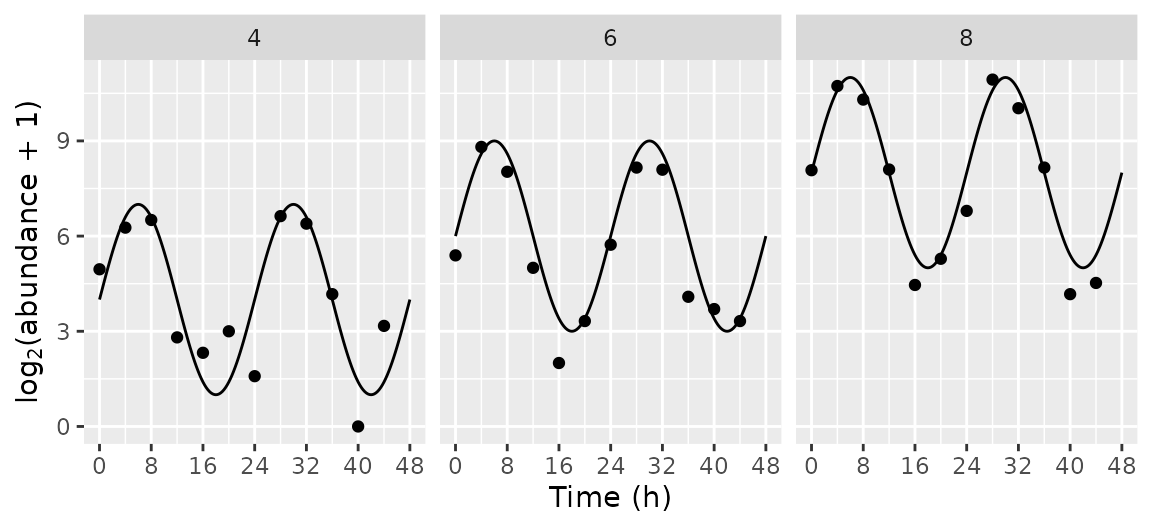## Poisson sampling at high resolution

featureGroups = data.table(amp = 1, base = 0,
rhyFunc = function(x) ifelse(x %% (2 * pi) < pi, 0.5, 4))

simData = simphony(featureGroups, timeRange = c(0, 24 * 4), interval = 0.1,
nReps = 1, nFeatures = 1, family = 'poisson')

mergedData = mergeSimData(simData)
expectedTrend = getExpectedAbund(simData$featureMetadata, sampleMetadata = simData$sampleMetadata)

ggplot() +
geom_line(data = expectedTrend, aes(x = time, y = mu)) +
geom_point(data = mergedData, aes(x = time, y = abund), shape = 21, size = 1) +
scale_x_continuous(breaks = seq(0, 24 * 4, 12)) +
labs(x = 'Time (h)', y = 'Abundance')